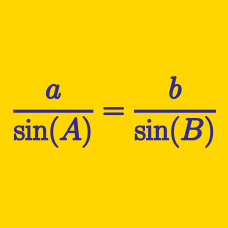Geometry

# Solving Triangles: Level 2 Challenges

If the angles of a triangle are in the ratio $$4:1:1,$$ then the ratio of the longest side to the perimeter is $$\text{__________}.$$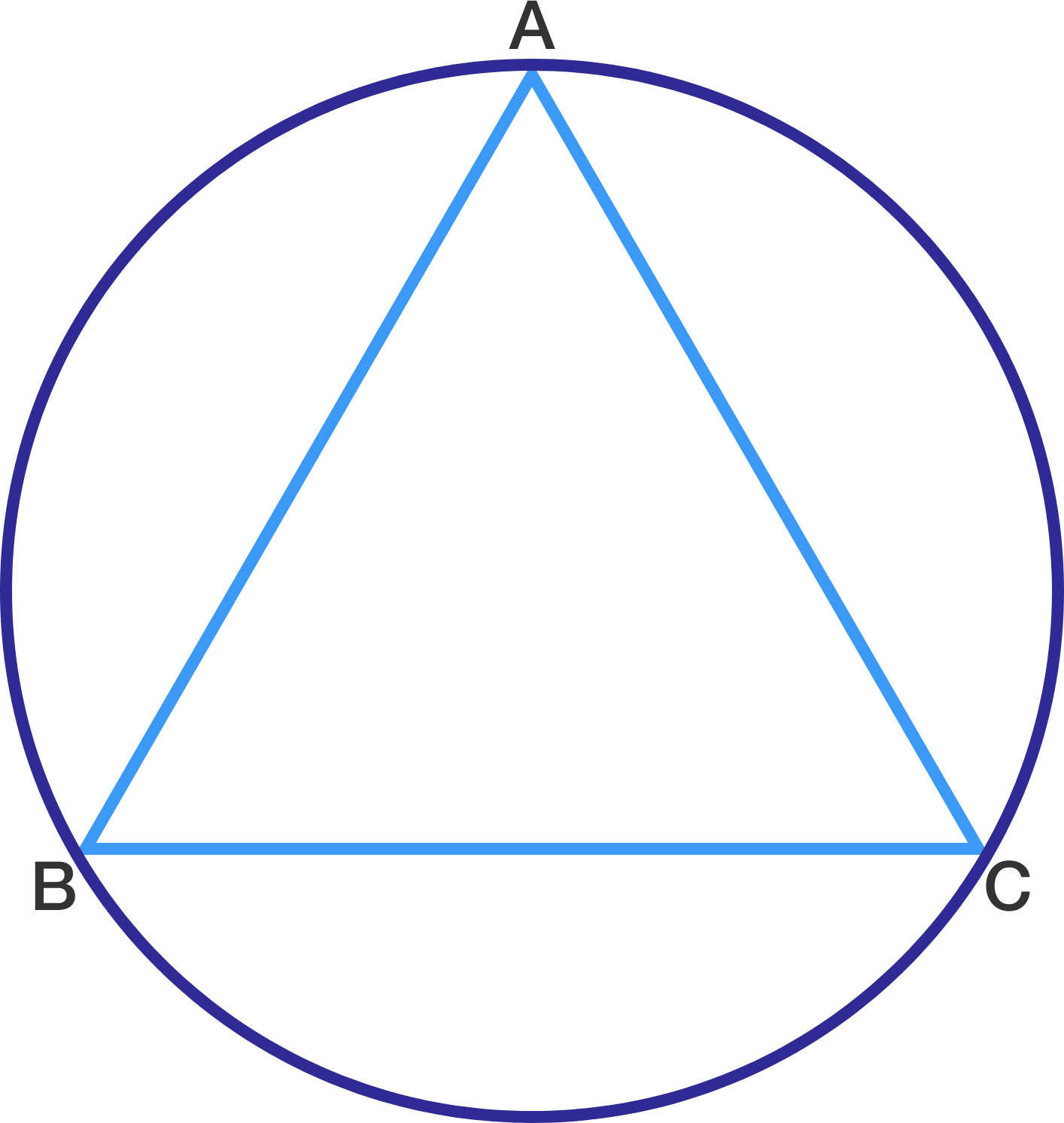In the figure, $$\triangle ABC$$ is an equilateral triangle where $$AB = 6$$ cm. The area of the circumcircle is $$k\pi$$ cm$$^{2}$$. Find the value of $$k$$.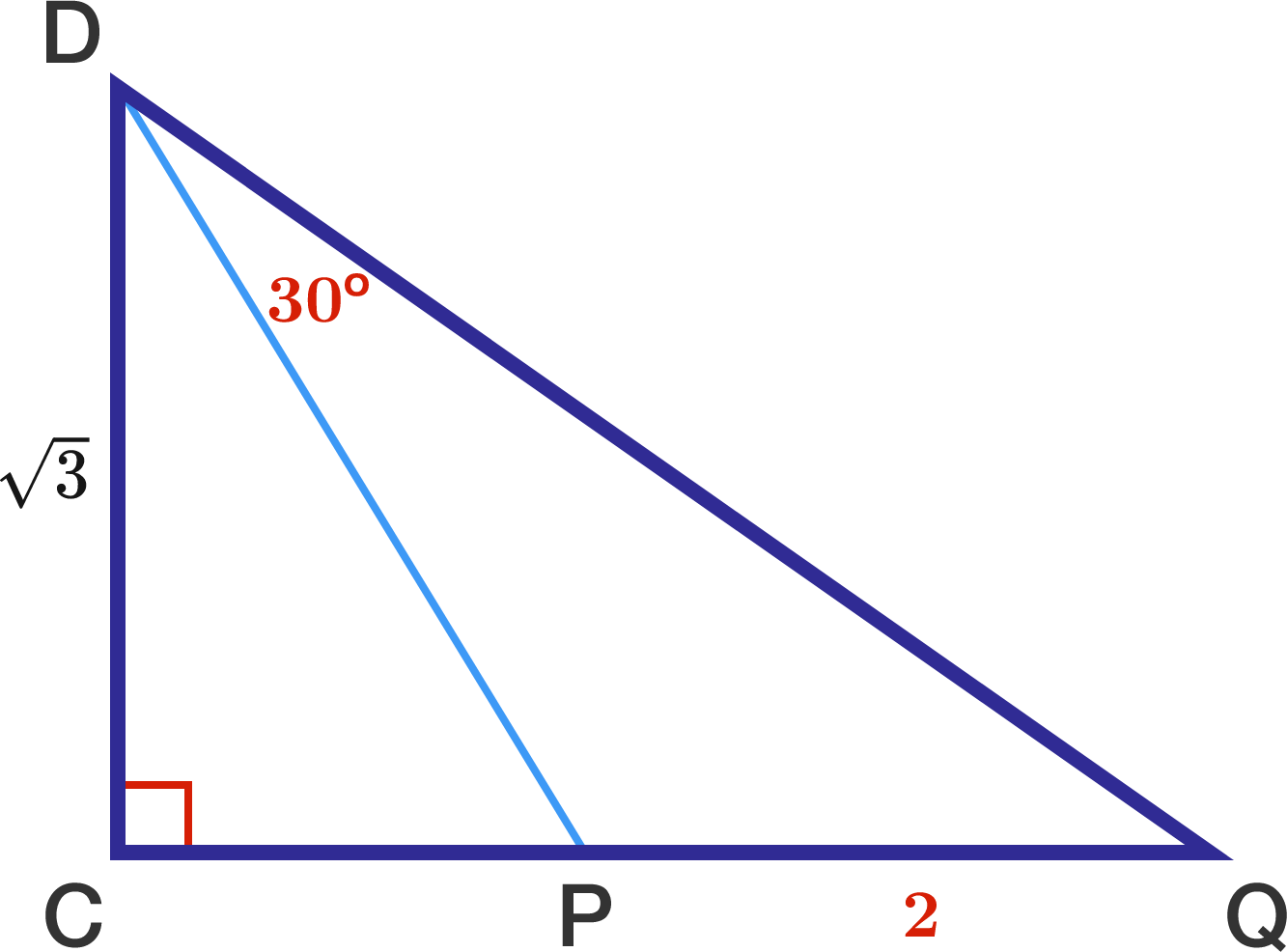If $$CD=\sqrt{3},$$ $$PQ=2,$$ and $$\angle PDQ=30^\circ,$$ find $$CP.$$

In triangle $$ABC$$, $$\angle BAC = 2 \angle ABC$$, $$AB= 31, AC = 13$$. What is $$BC^2$$?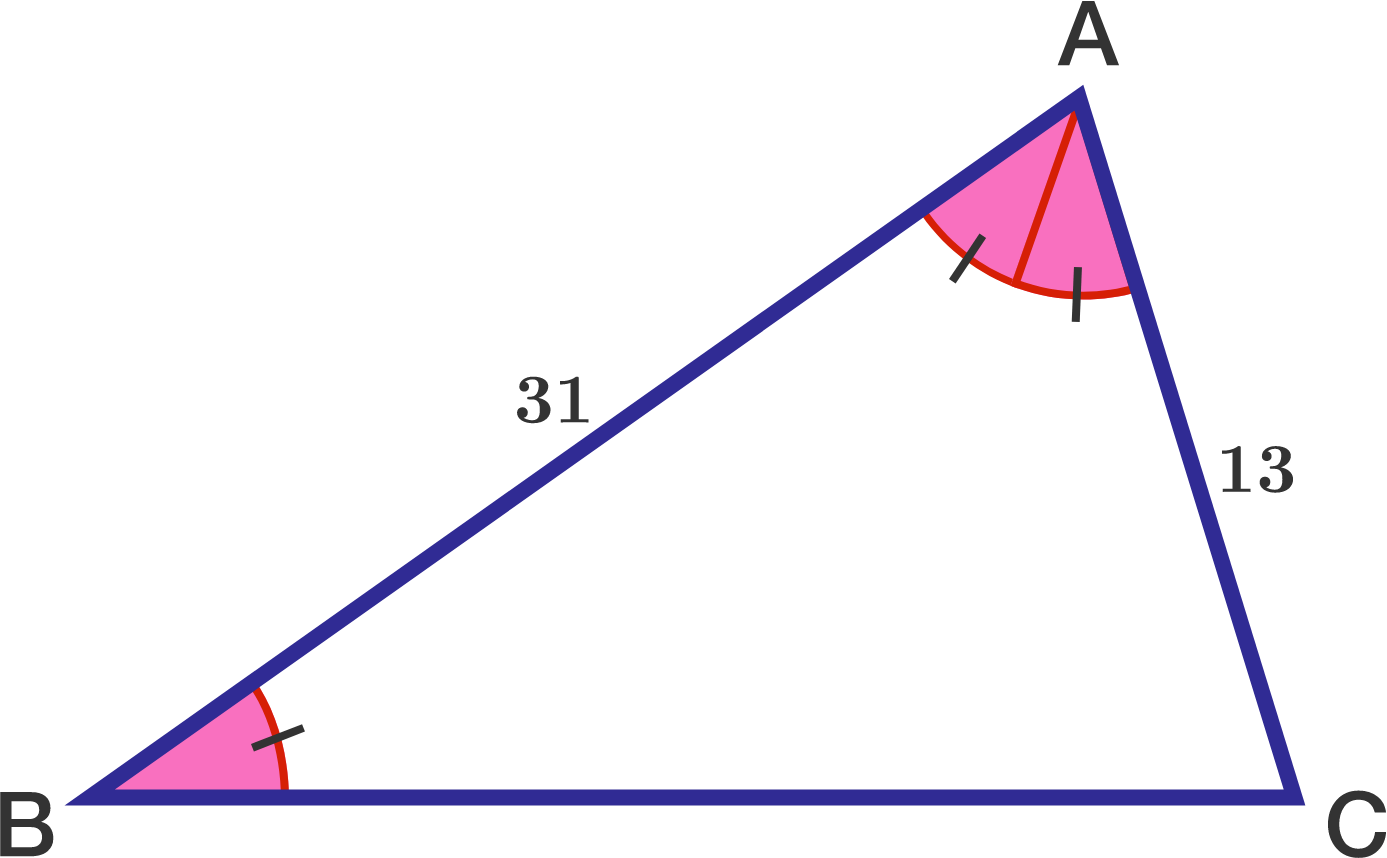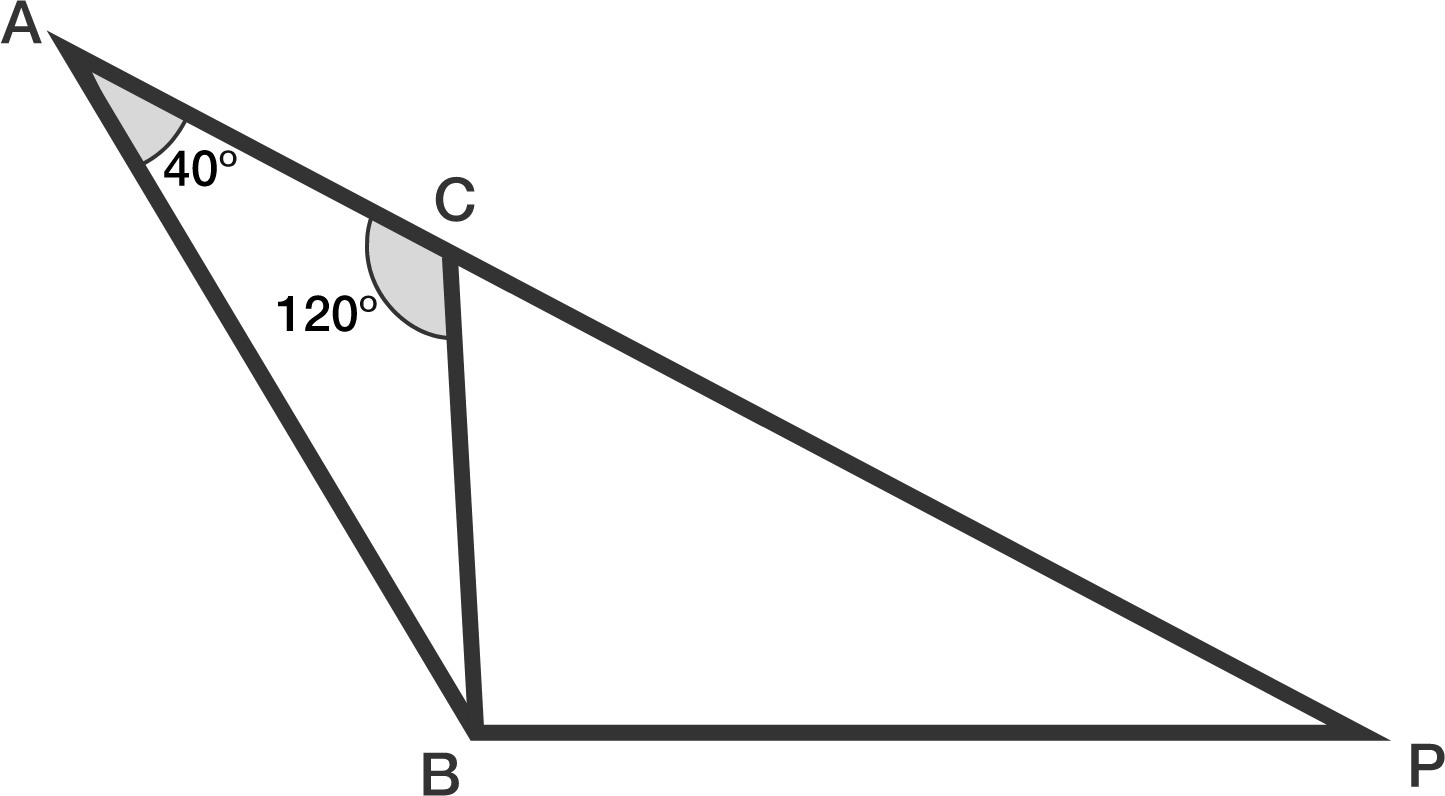If $$AP = AC + 2\cdot CB$$, what is the measure $$\angle ABP$$ in degrees?

×

Problem Loading...

Note Loading...

Set Loading...